# Simple and compound interest

## 0. Quick guide

This is a summary of the simple and compound interest materials used in the xlf presentation series. It incorporates algebraic formulae, examples, and Excel equations and functions where available. Excel FUNCTION syntax is provided, with example specific parameter settings in the details column.

### Symbols

• $\cdot \; (dot)$: multiplication
• $FV$: future value
• $I$: accrued interest
• $n$: number of periods
• $pa$: per annum
• $PV$: present value
• $r$: interest rate

## 1. Simple interest

• Amount of accumulated or accrued interest: $I=PV \cdot r \cdot n$
• Future value: $FV=PV(1+r \cdot n)$
• Present value: $PV=FV(1 + r \cdot n)^{-1}= FV / (1 + r \cdot n)$

Example: Bank deposit of $100,000 at 3% pa for 10 years. Interest paid to a non interest bearing (NIB) account. • 1.1 Simple interest, accrued: = 100,000 x 0.03 x 10 = 30,000 • Excel formula: =100000*3%*10 returns 30000 • Excel function: =ACCRINT(DATE(2014,1,1),DATE(2015,1,1),DATE(2024,1,1),3%,100000,1,3,TRUE) returns 30000. See figures 1 and 2 for the fx view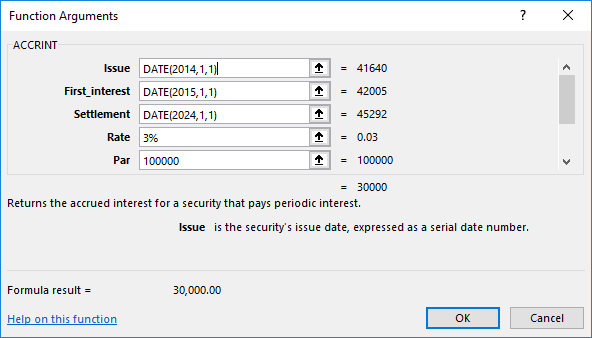Fig 1: Simple interest 1.1 screen 1 - ACCRINT function, parameters 1 to 5. Parameters 1 to 3 (Issue, First_interest, and Settlement) use a nested DATE function to convert yyyy,mm,yy to a date serial number (2014,1,1 >> 41640)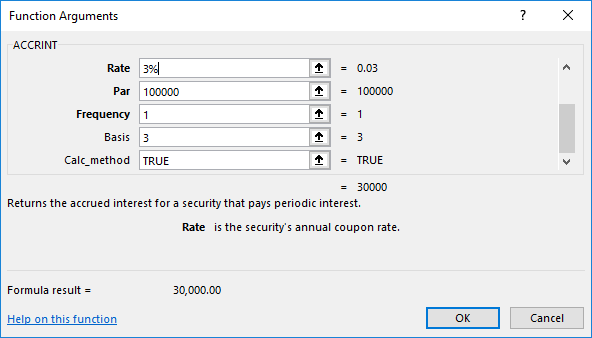Fig 2: Simple interest 1.1 screen 2 - ACCRINT function, parameters 4 to 8. Parameters 7 and 8 are optional as indicated by the non bold name. Basis 3 specifies the Australian Actual/365. calc_method is a Boolean logical value and is set to TRUE. This returns the total accrued interest from Issue date to Settlement date. • 1.2 Future value (principal plus interest): = 100,000 x (1 + 0.03 x 10) = 130,000 • Excel formula: =100000*(1+3%*10) returns 130000 • Excel formula: =100000*(1+0.03*10) returns 130000 • 1.3 Present value: = 130,000 / (1 + 0.03 x 10) = 100,000 • Excel formula: =130000/(1+3%*10) returns 100000 • Excel formula: =130000*(1+3%*10)^-1 returns 100000 The ACCRINT function returns the accrued interest for a security that pays periodic interest. Syntax: ACCRINT(issue,first_interest,settlement,rate,par,frequency,[basis],[calc_method]) argument / parameter details issue required the security's issue date as a date reference. In this case the deposit is assumed to be made on 1 January 2014. We use the Excel DATE function (Syntax DATE(YEAR,MONTH,DAY)) to return the sequential serial number for that particular, namely 41640. Based on the Excel for Windows 1900 date system. first_interest required the security's first interest date as a date reference. One year later, on 1 January 2015; serial number 42005. settlement required the security's settlement date as a date reference. The security settlement date is the date after the issue date when the security is traded to the buyer or the investment matures. In this case the deposit matures 10 years after deposit, 1 January 2024; serial number 45292. rate required the security's annual coupon interest rate. Set at 3% pa. par required the security's face value. If you omit par, ACCRINT uses$1,000. Note: par is listed as "required", but can be treated as an optional argument. In this case par is 100,000.
frequency required the number of coupon payments per year. For annual payments, frequency = 1; for semiannual, frequency = 2; for quarterly, frequency = 4. Interest is paid annually so FREQUENCY = 1.
[basis] optional the type of day count basis to use. A basis of 3 specifies the Australian Actual/365 basis system. In other words, 365 / 365 in this annual context.
[calc_method] optional a logical value that specifies the way to calculate the total accrued interest when the date of settlement is later than the date of first_interest. A value of TRUE (1) returns the total accrued interest from issue to settlement. A value of FALSE (0) returns the accrued interest from first_interest to settlement. The default value is TRUE. The deposit earns interest from the deposit date, so value is TRUE.

## 2. Compound interest

• Amount of accumulated interest: $I=PV((1+r)^n-1)$
• Future value: $FV=PV(1+r)^n$
• Present value: $PV=FV(1+r)^{-n}=FV/(1+r)^n$

Example: Bank deposit of $100,000 at 3% pa for 10 years. Interest credited to the account. • 2.1 Compound interest accrued: = 100,000((1 + 0.03)^10 - 1)) = 34,391.64 • Excel formula: =100000*((1+3%)^10-1) returns 34391.64 • Excel function: =FV(3%,10,0,-100000,1)-100000 returns 34391.64 • 2.2 Future value: = 100,000((1 + 0.03)^10)) = 134,391.64 • Excel formula: =100000((1+0.03)^10)) returns 134391.64 • Excel function: =FV(3%,10,0,-100000,1) returns 134391.64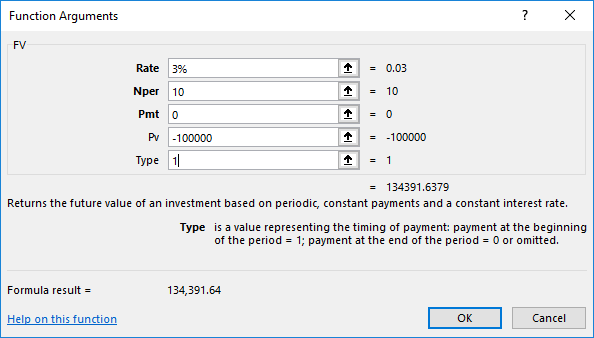Fig 3: Compound interest 2.1 and 2.2 - FV function: returns the future value of an annuity. Parameter #3 (Pmt) is set to 0 (zero) and the present value (Pv) -$100,000 is the cash flow to the account a time 0. Type 1 represents cash flows at the beginning of each period. In this example the setting for Type (0 for an ordinary annuity, or 1 for an annuity due) makes no difference as there is no annuity amount
• 2.3 Present value: = 134,391.64(1 + 0.03)^-10) = 100,000
• Excel formula: =134391.64*(1+0.03)^-10 returns 100000
• Excel formula: =134391.64/((1+0.03)^10) returns 100000
• Excel function: =PV(3%,10,0,134391.64,1) returns -100000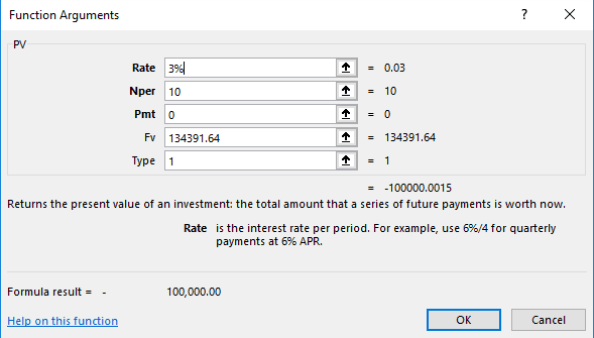Fig 4: Compound interest 2.3 - PV function: returns the present value of an annuity. Parameter #3 (Pmt) is set to 0 (zero) and the future value (Fv) 134391.64 is the cash flow received at the end of time (period) 10.
The FV function Returns the future value of an investment based on periodic, constant payments and a constant interest rate. Syntax: : FV(rate,nper,pmt,[pv],[type])
argument / parameter details
rate required The interest rate per period. 3% per year.
nper required the total number of payment periods in an annuity. The deposit spans 10 yearly periods, so nper = 10.
pmt required the payment made each period; it cannot change over the life of the annuity. Typically, pmt contains principal and interest but no other fees or taxes. If pmt is omitted, you must include the pv argument No extra deposits are made after the initial $100,000, so PMT is set to 0. pv optional the present value, or the lump-sum amount that a series of future payments is worth right now. If pv is omitted, it is assumed to be 0 (zero), and you must include the pmt argument. The initial lump sum deposit is$100,000. This is a cash outflow from the depositor's perspective, so PV is set to -100000.
type optional the number 0 or 1 and indicates when payments are due. If type is omitted, it is assumed to be 0. type = 1 indicates the beginning of the period.

The PV function returns the present value of an investment based on periodic, constant payments and a constant interest rate. Syntax: : PV(rate,nper,pmt,[fv],[type])
argument / parameter details
rate required The interest rate per period. 3% per year.
nper required The total number of payment periods in an annuity. The deposit spans 10 yearly periods, thus nper = 10.
pmt required The payment made each period; it cannot change over the life of the annuity. Typically, pmt contains principal and interest but no other fees or taxes. If pmt is omitted, you must include the pv argument. Set to 0.
fv optional The future value, or a cash balance that you want to have after the last payment is made. If fv is omitted, it is assumed to be 0 (zero), and you must include the pmt argument. The accumulated value of principal and interest at the end of 10 years is $134,391.64. This is the fv amount. type optional The number 0 or 1 and indicates when payments are due. If type is omitted, it is assumed to be 0. Deposits are assumed to occur at the beginning of each period. Type = 1. ## Comparison The gain to the depositor from compound interest is:$134,391.64 - $130,000.00 =$4,391.64.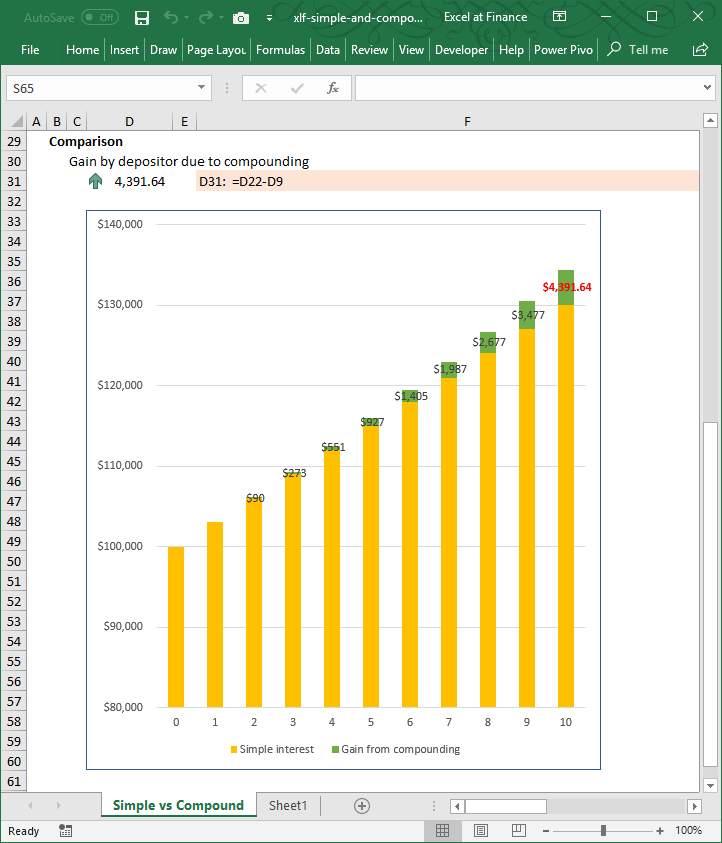Fig 6: Gains from compounding - \$100,000 at 3% p.a. for 10 years - compound annually

• Published: 16 July 2014
• Revised: Monday 10th of February 2020 - 11:10 AM, [Australian Eastern Standard Time (EST)]